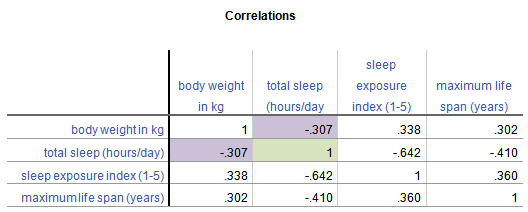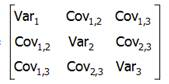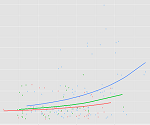# multilevel model

### The Difference Between Random Factors and Random Effects

January 9th, 2019 by

Mixed models are hard.

They’re abstract, they’re a little weird, and there is not a common vocabulary or notation for them.

But they’re also extremely important to understand because many data sets require their use.

Repeated measures ANOVA has too many limitations. It just doesn’t cut it any more.

One of the most difficult parts of fitting mixed models is figuring out which random effects to include in a model. And that’s hard to do if you don’t really understand what a random effect is or how it differs from a fixed effect. (more…)

### Three Issues in Sample Size Estimates for Multilevel Models

November 30th, 2012 by

If you’ve ever worked with multilevel models, you know that they are an extension of linear models. For a researcher learning them, this is both good and bad news.

The good side is that many of the concepts, calculations, and results are familiar. The down side of the extension is that everything is more complicated in multilevel models.

This includes power and sample size calculations. (more…)

### Sample Size Estimates for Multilevel Randomized Trials

May 1st, 2012 by

If you learned much about calculating power or sample sizes in your statistics classes, chances are, it was on something very, very simple, like a z-test.

But there are many design issues that affect power in a study that go way beyond a z-test.  Like:

• repeated measures
• clustering of individuals
• blocking
• including covariates in a model

Regular sample size software can accommodate some of these issues, but not all.  And there is just something wonderful about finding a tool that does just what you need it to.

Especially when it’s free.

Enter Optimal Design Plus Empirical Evidence software. (more…)

### Covariance Matrices, Covariance Structures, and Bears, Oh My!

September 16th, 2011 by

Of all the concepts I see researchers struggle with as they start to learn high-level statistics, the one that seems to most often elicit the blank stare of incomprehension is the Covariance Matrix, and its friend, the Covariance Structure.

And since understanding them is fundamental to a number of statistical analyses, particularly Mixed Models and Structural Equation Modeling, it’s an incomprehension you can’t afford.

So I’m going to explain what they are and how they’re not so different from what you’re used to.  I hope you’ll see that once you get to know them, they aren’t so scary after all.

### What is a Covariance Matrix?

There are two concepts inherent in a covariance matrix–covariance and matrix.  Either one can throw you off.

Let’s start with matrix.  If you never took linear algebra, the idea of matrices can be frightening.  (And if you still are in school, I highly recommend you take it.  Highly).  And there are a lot of very complicated, mathematical things you can do with matrices.

But you, a researcher and data analyst, don’t need to be able to do all those complicated processes to your matrices.  You do need to understand what a matrix is, be able to follow the notation, and understand a few simple matrix processes, like multiplication of a matrix by a constant.

The thing to keep in mind when it all gets overwhelming is a matrix is just a table.  That’s it.

A Covariance Matrix, like many matrices used in statistics, is symmetric.  That means that the table has the same headings across the top as it does along the side.

The simplest example, and a cousin of a covariance matrix, is a correlation matrix.  It’s just a table in which each variable is listed in both the column headings and row headings, and each cell of the table (i.e. matrix) is the correlation between the variables that make up the column and row headings.  Here is a simple example from a data set on 62 species of mammal:From this table, you can see that the correlation between Weight in kg and Hours of Sleep, highlighted in purple, is -.307. Smaller mammals tend to sleep more.

You’ll notice that this is the same above and below the diagonal. The correlation of Hours of Sleep with Weight in kg is the same as the correlation between Weight in kg and Hours of Sleep.

Likewise, all correlations on the diagonal equal 1, because they’re the correlation of each variable with itself.

If this table were written as a matrix, you’d only see the numbers, without the column headings.

### Now, the Covariance Matrix

A Covariance Matrix is very similar. There are really two differences between it and the Correlation Matrix. It has this form:First, we have substituted the correlation values with covariances.

Covariance is just an unstandardized version of correlation.  To compute any correlation, we divide the covariance by the standard deviation of both variables to remove units of measurement.  So a covariance is just a correlation measured in the units of the original variables.Covariance, unlike  correlation, is not constrained to being between -1 and 1. But the covariance’s sign will always be the same as the corresponding correlation’s. And a covariance=0 has the exact same meaning as a correlation=0: no linear relationship.

Because covariance is in the original units of the variables, variables on scales with bigger numbers and with wider distributions will necessarily have bigger covariances. So for example, Life Span has similar correlations to Weight and Exposure while sleeping, both around .3.

But values of Weight vary a lot (this data set contains both Elephants and Shrews), whereas Exposure is an index variable that ranges from only 1 to 5. So Life Span’s covariance with Weight (5113.27) is much larger than than with Exposure (10.66).

Second, the diagonal cells of the matrix contain the variances of each variable. A covariance of a variable with itself is simply the variance. So you have a context for interpreting these covariance values.

Once again, a covariance matrix is just the table without the row and column headings.

Covariance Structures are just patterns in covariance matrices.  Some of these patterns occur often enough in some statistical procedures that they have names.

You may have heard of some of these names–Compound Symmetry, Variance Components, Unstructured, for example.  They sound strange because they’re often thrown about without any explanation.

But they’re just descriptions of patterns.

For example, the Compound Symmetry structure just means that all the variances are equal to each other and all the covariances are equal to each other. That’s it.

It wouldn’t make sense with our animal data set because each variable is measured on a different scale. But if all four variables were measured on the same scale, or better yet, if they were all the same variable measured under four experimental conditions, it’s a very plausible pattern.

Variance Components just means that each variance is different, and all covariances=0. So if all four variables were completely independent of each other and measured on different scales, that would be a reasonable pattern.

Unstructured just means there is no pattern at all.  Each variance and each covariance is completely different and has no relation to the others.

There are many, many covariance structures.  And each one makes sense in certain statistical situations.  Until you’ve encountered those situations, they look crazy.  But each one is just describing a pattern that makes sense in some situations.

### How to Combine Complicated Models with Tricky Effects

July 22nd, 2011 by

Need to dummy code in a Cox regression model?Interpret interactions in a logistic regression?This is where statistical analysis starts to feel really hard. You’re combining two difficult issues into one.

You’re dealing with both a complicated modeling technique at Stage 3 (survival analysis, logistic regression, multilevel modeling) and tricky effects in the model (dummy coding, interactions, and quadratic terms).

The only way to figure it all out in a situation like that is to break it down into parts.  (more…)

### When NOT to Center a Predictor Variable in Regression

February 9th, 2009 by

There are two reasons to center predictor variables in any type of regression analysis–linear, logistic, multilevel, etc.

1. To lessen the correlation between a multiplicative term (interaction or polynomial term) and its component variables (the ones that were multiplied).

2. To make interpretation of parameter estimates easier.

I was recently asked when is centering NOT a good idea? (more…)